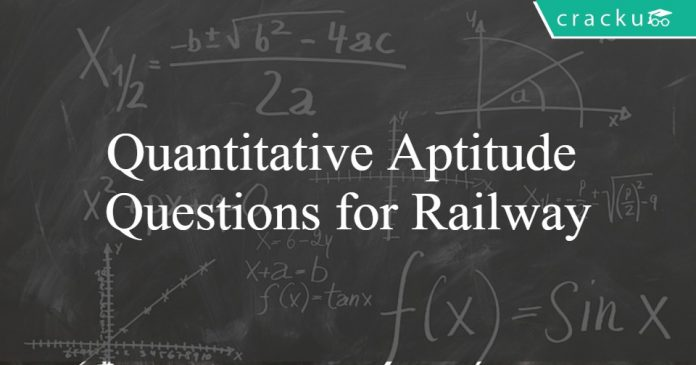# Quantitative Aptitude Questions for Railway Group-D and RRB ALP Exams

1
10500Quantitative Aptitude Questions for Railway

Quantitative Aptitude Questions for Railway exams:

Railway Quantitative aptitude maths questions for RRB ALP and Group-D exams. Important questions and answers on RRB quant. Download Practice questions PDF for Railway exams.

Download Railways preparation App

Question 1: Find the next number in the following number series. 3,5,10,17,29,48 …………

a) 82
b) 72
c) 77
d) 79

Question 2: Choose the odd one in the following

a) 3
b) 5
c) 7
d) 9

Question 3: If the following numbers are arranged in descending order; the middle digit of the middle number will be 687, 692, 598, 482, 767

a) 6
b) 3
c) 8
d) 5

Question 4: 12 copies of a book were sold for Rs. 1800, thereby gaining the cost price of 3 copies. The cost price of a copy is

a) Rs. 120
b) Rs. 150
c) Rs. 1200
d) Rs. 1500

Question 5: A sum of Rs. 625 is made up of 80 currency notes which are of either Rs. 10 or Rs. 5 denomination. The number of Rs. 10 notes are

a) 35
b) 45
c) 40
d) 30

Question 6: A certain job was assigned to a group of men to do in 20 days. But 12 men did not turn up for the job and the remaining men did the job in 32 days. The original number of men in the group was

a) 32
b) 34
c) 36
d) 40

Question 7: A certain number of men can do a work in 60 days. If there were 8 men more it could be finished in 10 days less. How many men were there in the beginning ?

a) 40
b) 35
c) 45
d) 50

Question 8: The average expenditure of a man for the first five months is Rs. 1200 and for the next seven months is Rs. 1300. Find his monthly average income if he saves Rs. 2900 during the year ?

a) Rs. 1500
b) Rs. 1475
c) RS. 1450
d) Rs. 1425

Question 9: The average temperature for monday, Tuesday and wednesday was 40°C. The
average for Tuesday, wednesday and Thursday was 41°C. If on Thursday temperature is 45°C, What was it on monday ?

a) 40°C
b) 41°C
c) 42°C
d) 43.5°C

Question 10: Of the three numbers, second is twice the first and is also thrice the third. If the average of the three numbers is 44, the largest number is

a) 24
b) 72
c) 36
d) 108

Solutions for Quantitative Aptitude Questions for Railway:

Solutions:

1) Answer (D)

The difference between the numbers is 2, 5, 7, 12, 19, etc
These numbers are in a sequence. The sum of the first two numbers forms the third number. The
sum of the second and third is the fourth number and so on. So, the next number is 31.
So the next number in the actual sequence is 31 + 48 = 79.

2) Answer (D)

Only 9 is the composite number in the given list. Others are all prime numbers.

3) Answer (C)

When arranged in the descending order the numbers are as follows:
767, 692, 687, 598, 482
So, the middle digit of the middle number is 8.

4) Answer (A)

SP of 12 books=1800
SP=CP + Profit
Given, Profit of 12 books=3CP
∴1800=12CP + 3CP
15CP=1800
CP=120

5) Answer (B)

Let there be x 10 rupee notes in the sum. The number of 5 rupee notes = 80 – x
The sum = 10x + (80 – x)* 5 = 625
So, x = 45

6) Answer (A)

Let there be x men initially. They were supposed to complete the work in 20 days.
When 12 men didn’t show up the work was done in 32 days
So, x- 12 men did the work in 32 days
But x-12 men were supposed to take 20x/ (x-12) days
So, 20 x/ (x-12) = 32
so,
x = 32

7) Answer (A)

Let there be m men originally to work in 60 days
If the men were m + 8, the work would be completed in 50 days.
(m+8)/m = 60/50
So, m = 40

8) Answer (A)

Let his monthly salary be x rupees.
So, 5 (x – 1200) + 7 (x-1300) = 2900
12x – 6000 – 9100 = 2900
So, x = 18000/12 = 1500

9) Answer (C)

Total temperature for Monday, Tuesday, Wednesday and Thursday = 40 x 3 + 45 = 165 C
Total for Tuesday, Wednesday and Thursday = 41 x 3 = 123 C
So, Temperature for Monday = 165 – 123 = 42 C

10) Answer (B)

Let the first number be 3x. Second number is 6x. Third number is 2x.
So average = (2x + 3x + 6x)/3 = 11x/3
But 11x/3 = 44
So, x = 12
6x = 72

This quantitative aptitude questions and answers for Railway exams will be useful for RRB ALP (Assistant Loco pilot) & technician and Group-D.